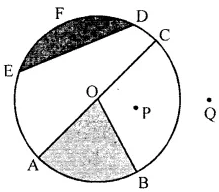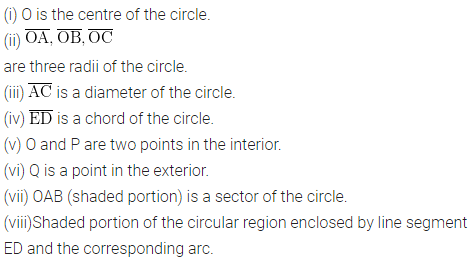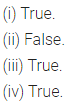ML Aggarwal Class 6 Solutions Chapter 10 Basic Geometrical Concept Ex 10.4 for ICSE Understanding Mathematics acts as the best resource during your learning and helps you score well in your exams.

## ML Aggarwal Class 6 Solutions for ICSE Maths Chapter 10 Basic Geometrical Concept Ex 10.4

Question 1.
In the given figure, identify:
(i) the centre of the circle
(iii) a diameter
(iv) a chord
(v) two points in the interior
(vi) a point in the exterior
(vii) a sector
(viii) a segmentSolution:Question 2.
State whether the following statements are true (T) or false (F):
(i) Every diameter of a circle is also a chord.
(ii) Every chord of a circle is also a diameter.
(iii) Two diameters of a circle will necessarily intersect.
(iv) The centre of the circle is always in its interior.
Solution: